# H2 Math Sun 930amMF26

Vectors Q7 [Homework] (i)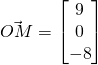Using ratio theorem,Sinceis perpendicular to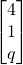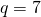(ii)
To be a parallelogram,(iii)
LetSince— (1)— (2)
Solving,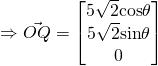Vectors Q8 [Homework] (i)(ii)
Let R be the top of the vertical pillar,Since R is collinear with A and C, R is the intersection of line AC and QR., and the height is 9m.

(iii)Vectors Q9 [Homework] (i)Normal of(ii)
Letbe the acute angle(iii)— (1)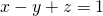— (2)

Using GC,(iv)
Let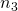be the normal ofLength of projection(v)
Required distance(vi)
Let normal ofIfintersect at l,nlies onNot readable? Change text.